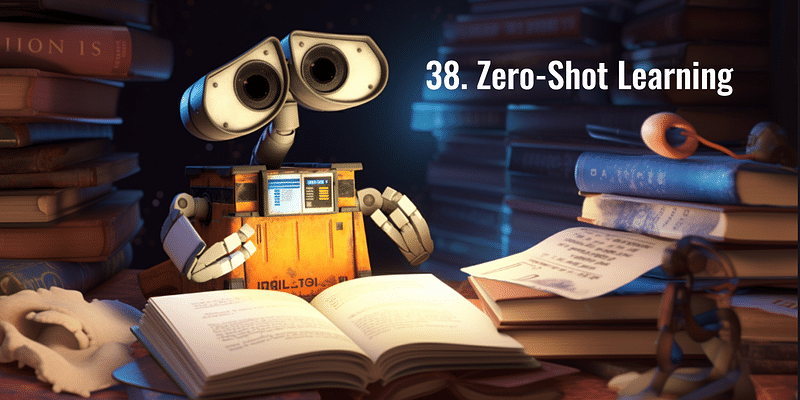# Mastering Backpropagation: Unlocking the Secrets of Neural Network Training

## Discover how backpropagation enables neural networks to learn and improve performance in AI. Dive into its step-by-step process.Friday June 16, 2023,

In the realm of artificial intelligence and machine learning, neural networks have proven to be a powerful tool for solving complex problems. These networks, inspired by the workings of the human brain, are composed of interconnected nodes or artificial neurons. Training a neural network involves adjusting the weights and biases associated with these neurons to optimize their performance. One of the fundamental algorithms used for this purpose is backpropagation.

Backpropagation, short for "backward propagation of errors," is an algorithm that lies at the heart of training neural networks. It enables the network to learn from its mistakes and make adjustments accordingly. The process involves propagating the errors backward through the network, hence the name.

### Forward Pass:

During the forward pass, the input data is fed into the neural network. Each neuron receives this input, performs a weighted sum of the inputs, adds a bias term, and applies an activation function to produce an output. This output is then passed as input to the next layer of neurons until the final output is generated.

### Calculating the Loss:

Once the output is generated, it is compared to the expected output, which is known during the training phase. The difference between the predicted and expected outputs is measured using a loss function, such as mean squared error or cross-entropy loss. The loss function quantifies how well the network is performing on the given task.

### Backward Pass:

The backward pass is where the magic happens. It involves computing the gradients of the loss function with respect to the weights and biases of the network. This is achieved using a technique called the chain rule from calculus. The gradients indicate the direction and magnitude of adjustments required to minimize the loss.

With the gradients calculated, the weights and biases of the network can be updated. This step follows an optimization algorithm, typically stochastic gradient descent (SGD). SGD adjusts the weights and biases in the direction opposite to the gradients, scaled by a learning rate. The learning rate determines the step size taken during the optimization process.

### Iterative Process:

Steps 1 to 4 are repeated for multiple iterations or epochs until the network converges or reaches a predefined stopping criterion. The network learns from each iteration, gradually reducing the loss and improving its performance on the task at hand.

Backpropagation has revolutionized the field of neural networks by enabling the training of deep learning models. Deep neural networks, composed of multiple hidden layers, have a greater capacity to learn complex patterns and extract high-level features from data. Backpropagation allows the gradients to be efficiently calculated across all layers of the network, making the training process feasible.

However, backpropagation is not without challenges. One of the primary issues is the vanishing or exploding gradient problem. As the gradients are propagated backward through the network, they can diminish or explode exponentially, making it difficult to update the weights effectively. Various techniques, such as weight initialization strategies and activation functions like ReLU, have been developed to mitigate these issues.

Backpropagation is a fundamental algorithm that enables neural networks to learn from their mistakes and improve their performance over time. It involves a forward pass, calculating the loss, propagating the errors backward, and updating the weights and biases of the network. While it has its challenges, backpropagation has paved the way for the success of deep learning and continues to be a key component in training neural networks for a wide range of tasks in the field of artificial intelligence.